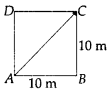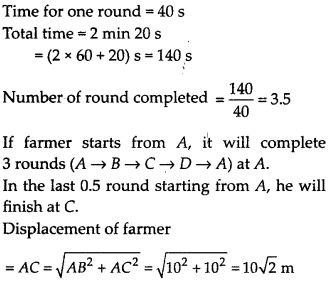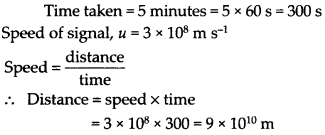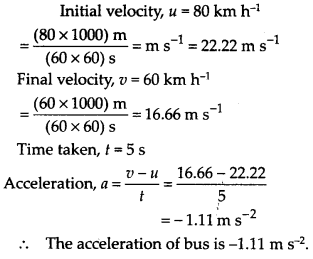• +91 9971497814
• info@interviewmaterial.com

# Chapter 8 Motion Interview Questions Answers

### Related Subjects

Question 1 :
An object has moved through a distance. Can it have zero displacement? If yes support your answer with an example.

Yes, an object moving through a distance can have zero displacement. This happens when final position of the object coincides with its initial position. Example : If an object travels from point A and reaches to the same point A, then its displacement is zero.

Question 2 :
A farmer moves along the boundary of a square field of side 10 m in 40 s. What will be the magnitude of displacement of the farmer at the end of 2 minutes 20 seconds?

Figure ABCD is a square field of side 10 m.Question 3 :
Which of the following is true for displacement?
(a) It cannot be zero.
(b) Its magnitude is greater than the distance travelled by the object.

(a) False
(b) False

Question 4 :
Distinguish between speed and velocity.

 Speed Velocity The distance travelled by a moving body per unit time is called its speed. The distance travelled by a moving body in a particular direction per unit time is called its velocity. It is a scalar quantity. It is a vector quantity.

Question 5 :
Under what condition(s) is the magnitude of average velocity of an object equal to its average speed?

The magnitude of average velocity of an object is equal to its average speed if the object moves in a straight line in a particular direction.

Question 6 :
What does.the odometer of ,an automobile measure?

The odometer of an automobile measures the distance travelled by a vehicle.

Question 7 :
What does the path of an object look like when it is in uniform motion?

In uniform motion, the path of an object can be a straight line, curved line or a circle. It can have any shape. This is because in uniform motion, speed is constant, the direction of motion may change.

Question 8 :
During an experiment, a signal from a spaceship reached the ground station in five minutes. What was the distance of the spaceship from the ground station? The signal travels at the speed of light, that is 3 × 108 m s-1.

Answer 8 :Question 9 :
When will you say a body is in

uniform acceleration
non-uniform acceleration

1. Uniform acceleration : When a body travels with thp same velocity in the given time, then the acceleration is said to be uniform.
2. Non-uniform acceleraiion : When a body moves with unequal velocity in the equal interval of time, the body is said to be moving with non-uniform acceleration.

Question 10 :
A bus decreases its speed from 80 km h’1 to 60 km h-1 in 5 s. Find the acceleration of the bus.

Answer 10 :Negative sign shows retardation.

Todays Deals### Chapter 8 Motion Contributorskrishan

Name:
Email:

# Latest News# 9000 interview questions in different categories